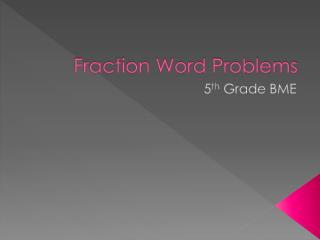Download PresentationFraction Word Problems

# Fraction Word Problems - PowerPoint PPT PresentationDownload Presentation## Fraction Word Problems

- - - - - - - - - - - - - - - - - - - - - - - - - - - E N D - - - - - - - - - - - - - - - - - - - - - - - - - - -
##### Presentation Transcript

1. Fraction Word Problems 5th Grade BME

2. Essential Question • How do I solve word problems involving fractions?

3. Overview • We are going to go step-by-step through the basics of solving word problems involving fractions. Pay attention. There will be a quiz!

4. School Fundraiser • You are whipping up your Uncle Bubba's famous honey chipotle BBQ sauce for a school fundraiser. The recipe calls for 3/4 cup of sugar. You need to make 5 batches of sauce. How much sugar do you need? • Find the important information. • You are trying to make 5 batches of sauce. • For each batch, you need ¾ cup. • What operation will we use?

5. School Fundraiser • Why would you use multiplication? • You would multiply because you are trying to find 5 times the amount of the original recipe. • How would you set up the equation? • 3/4 x 5= • What is the denominator for 5? • Now, let’s solve- 3/4 x 5/1. • To multiply fractions, we multiply straight across. • 3 x 5 = 15 • 4 x 1 = 4 • 15/4 is the product.

6. School Fundraiser • 15/4 is an improper fraction. • Improper fractions are fractions in which the numerator is greater than the denominator. While, we may need improper fractions from time to time, they are not considered to be in “simplest form.” • We know the fraction bar is also a division sign. So, let’s simplify this fraction.

7. School Fundraiser • 15 ÷ 4 = 3 R 3 • 3 is our whole number. We then place the remainder over our original denominator (4). We now have a mixed number, 3 ¾. The fraction is now in simplest form. • You will need 3 ¾ cups of sugar.

8. Hair Dye • You decide to dye your hair various colors for Halloween. If you dye of it 1/3 blue, 1/4 pink, and 1/4 black, how much of your original hair color is left? • Hint, just because you see “of” here, you may not need to multiply. Since you don’t have a number to multiply by, you should try a different operation.

9. Hair Dye • What did you decide to do first? • We know that fractions show us parts of a whole. • We are trying to determine what fraction of the whole (head) was NOT dyed for Halloween. • What do we need to figure out first? • First, we need to determine how much of the head IS dyed. • To do so, you would add the given fractions.

10. Hair Dye • Remember to add and subtract fractions, you must have common denominators. You use LCM to find the LCD. • 1/3 + 1/4 + 1/4 = • What would be the LCD? • 12 • Now, let’s change our fractions! • 4/12 + 3/12 + 3/12 = 10/12 • 10/12 is NOT in simplest form.

11. Hair Dye • How can we simplify 10/12? • We must find the GCF. • Factors of 10: 1, 10, 2, 5 • Factors of 12: 1, 12, 2, 6, 3, 4 • GCF= 2 • Divide the numerator and the denominator by 2. • The fraction in simplest form is 5/6.

12. Hair Dye • The problem asked how much of the head is NOT dyed. • Remember the numerator shows us the part, while the denominator shows us the whole. • 5/6 + ? = 6/6 • 6/6 – 5/6 = 1/6 • 1/6 of the head was NOT dyed.

13. Ribbon Cutting • How many 2 ¾ feet long strips of ribbon can be cut from a ribbon that is 7 ½ feet long? • What do we know? • We have a ribbon that is 7 ½ feet long. • We want to cut it up into pieces that are 2 ¾ feet long. • Cutting it up into pieces is a signal to divide. • **Dividing Mixed Numbers- Change into improper fractions first. You will simplify at the end.

14. Ribbon Cutting • Changing mixed numbers to improper fractions. • Step One: Multiply the denominator and the whole number. • Step Two: Add the numerator to the product from step one. • Step Three: Place the new numerator on top of the original denominator.

15. Ribbon Cutting • 7 ½ = 15/2 • 2 ¾ = 11/4 • Now that you have improper fractions, it is time to divide. • Remember: Keep, Change, Flip. • We are dividing up the 7 ½ feet of ribbon. • Set your equation up like so: • 15/2 ÷ 11/4

16. Ribbon Cutting • Keep-Change-Flip • 15/2 (keep) ÷ (change) 11/4 (flip) • New equation 15/2 x 4/11 = • 30/22 • Now simplify • 30 ÷ 22 = 1 R 8 • 30/22 = 1 8/30 • Simplest form= 1 4/15 • You could cut 1 4/15 strips of ribbon.